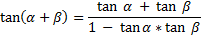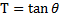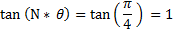Statement of Purpose for Part VII

# An Approach to Mathematic Functions Basics

## Section XL - A Statement of Purpose for Part VII

### A Statement of Purpose for Part VII :

• In this Part VII of the paper , we shall take a close look at :

• the Tangent Addition Formula :

•### Flow Chart for Part VII :

• In Section XLI coming up next :

• We shall go thru the derivation process for the Tangent Addition Formula .

• In Section XLII :

• We shall take a quick look at the expression for :

• the { Tangent of [ N * Theta ] } expressed in terms of the { Tangent of [ Theta ] } .

• In Section XLIII :

• We shall identify the co-efficients in the afore-mentioned expression above :

• as being members of the same row in the Pascal Triangle .

• In Section XLIV :

• We shall set up the Algebraic Equation to solve for :

• the value of [ T ] in :

•when :

•